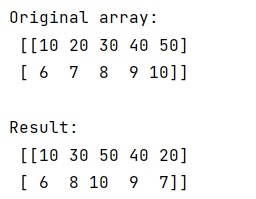# Rearrange columns of NumPy 2D array

Learn, how to import PNG files into NumPy in Python?
Submitted by Pranit Sharma, on January 18, 2023

NumPy is an abbreviated form of Numerical Python. It is used for different types of scientific operations in python. Numpy is a vast library in python which is used for almost every kind of scientific or mathematical operation. It is itself an array which is a collection of various methods and functions for processing the arrays.

## NumPy Array - Rearranging columns of a 2D array

Suppose that we are given a 2-dimensional NumPy array and we need to change the order of this array to a new arbitrary order.
We need to apply this change in such a way that the first column of the original stays in place, the second moves to the last column, and so on.

For this purpose, we will simply use the fancy indexing technique. We will simply modify the array by assigning the new permuted indices to this array.

Let us understand with the help of an example,

## Python code to rearrange columns of NumPy 2D array

```# Import numpy
import numpy as np

# Creating a numpy array
arr = np.array([
[10, 20, 30, 40, 50],
[6,  7,  8,  9,  10]
])

# Display original array
print("Original array:\n",arr,"\n")

# Rearranging order of array
res = arr[:, [0, 2, 4, 3, 1]]

# Display result
print("Result:\n",res)
```

Output:What's New (MCQs)

Top Interview Coding Problems/Challenges!

IncludeHelp's Blogs

Languages: » C » C++ » C++ STL » Java » Data Structure » C#.Net » Android » Kotlin » SQL
Web Technologies: » PHP » Python » JavaScript » CSS » Ajax » Node.js » Web programming/HTML
Solved programs: » C » C++ » DS » Java » C#
Aptitude que. & ans.: » C » C++ » Java » DBMS
Interview que. & ans.: » C » Embedded C » Java » SEO » HR
CS Subjects: » CS Basics » O.S. » Networks » DBMS » Embedded Systems » Cloud Computing
» Machine learning » CS Organizations » Linux » DOS
More: » Articles » Puzzles » News/Updates